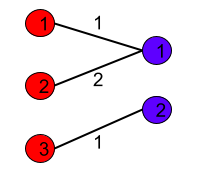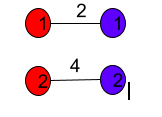Problem title
Difficulty
Avg time to solve

Reverse A LL
Moderate
30 mins
Remove K Corner Elements
Easy
15 mins
Print All Subsets
Easy
20 mins
Minimum Cost To Connect Two Groups Of Points
Hard
15 mins
BST to sorted DLL
Moderate
50 mins
Reverse String Word Wise
Easy
--
Squares of a Sorted Array
Easy
15 mins
Flood Fill Algorithm
Moderate
10 mins
Nth Fibonacci Number
Easy
--
Maximum of All Subarrays of Size K
Easy
15 mins16

# Minimum Cost To Connect Two Groups Of Points

Difficulty: HARD
Avg. time to solve
15 min
Success Rate
85%

Problem Statement

#### Now you are supposed to find the minimum cost it takes to connect the two groups.

##### Input Format :
``````The first line contains an integer ‘T’ denoting the number of test cases. Then each test case follows.

The first input line of each test case contains two integers ‘N’ and ‘M’, denoting the number of rows and columns of the “cost” matrix.

Each of the next ‘N’ lines contains ‘M’ space-separated integers denoting the elements of the “cost” matrix.
``````
##### Output Format :
``````For each test case, print an integer denoting the minimum cost it takes to connect the two groups.

Print the output of each test case in a separate line.
``````
##### Note :
``````You are not required to print the expected output; it has already been taken care of. Just implement the function.
``````
##### Constraints :
``````1 <= T <= 5
1 <= N <= 10
1 <= M <= 10
0 <= cost[i][j] <= 100

Time limit: 1 sec
``````
##### Sample Input 1 :
``````2
3 2
1 2
2 3
4 1
2 2
2 5
3 4
``````
##### Sample Output 1 :
``````4
6
``````
##### Explanation of Sample Output 1 :
``````For the first test case, the optimal way of connecting the group is as following:
````````````The minimum cost will be 4.

For the second test case, the optimal way of connecting the group is as following:
````````````The minimum cost will be 6.
``````
##### Sample Input 2 :
``````2
1 1
5
1 3
1 2 3
``````
##### Sample Output 2 :
``````5
6
``````
##### Explanation of Sample Output 2 :
``````For the first test case, there is only one possible way of connection and its cost is 5.

For the second test case, the minimum cost will be 6.
``````Console